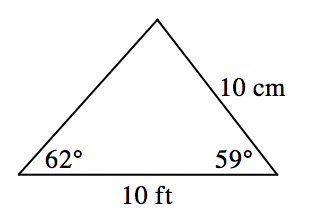### Home > INT2 > Chapter 1 > Lesson 1.3.4 > Problem1-111

1-111.

Examine each diagram below. Which diagrams are possible? Which are impossible? Justify each conclusion.

What properties do you know about the sides and angles in triangles?

1.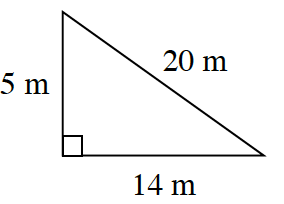Since the figure in part (a) is a right triangle, it must satisfy the Pythagorean Theorem.

1.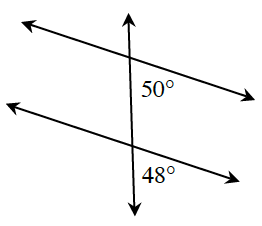Possible (the two lines are not marked as parallel).

1.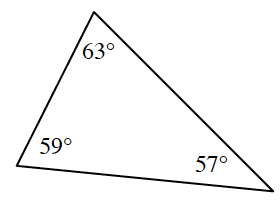The sum of angles in a triangle is always $180^\circ$.

1.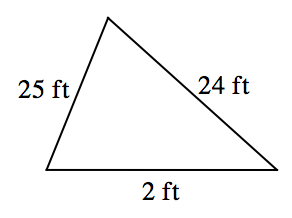1.